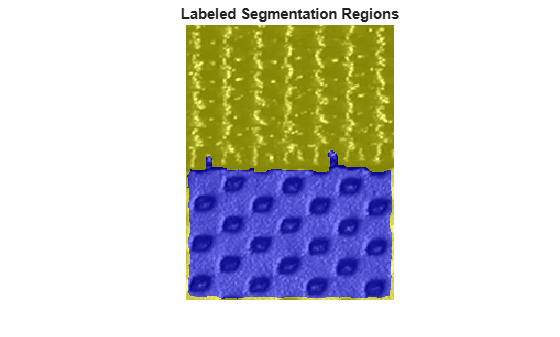Documentation

This is machine translation

Mouseover text to see original. Click the button below to return to the English version of the page.

Texture Segmentation Using Texture Filters

This example shows how to use texture segmentation to identify regions based on their texture. Your goal is to segment two kinds of fabric in an image using texture filters.

Read in `bag.png`.

```I = imread('bag.png'); figure imshow(I)```Step 2: Create Texture Image

Use `entropyfilt` to create a texture image. The function `entropyfilt` returns an array where each output pixel contains the entropy value of the 9-by-9 neighborhood around the corresponding pixel in the input image `I`. Entropy is a statistical measure of randomness.

`E = entropyfilt(I);`

Use `rescale` to rescale the texture image `E` so that its values are in the default range for a double image.

```Eim = rescale(E); figure imshow(Eim)```Step 3: Create Rough Mask for the Bottom Texture

Threshold the rescaled image `Eim` to segment the textures. A threshold value of 0.8 is selected because it is roughly the intensity value of pixels along the boundary between the textures.

```BW1 = imbinarize(Eim, .8); imshow(BW1);``````figure imshow(I)```The segmented objects in the binary image `BW1` are white. If you compare `BW1` to `I`, you notice the top texture is overly segmented (multiple white objects) and the bottom texture is segmented almost in its entirety. You can extract the bottom texture using `bwareaopen`.

```BWao = bwareaopen(BW1,2000); imshow(BWao)```Use `imclose` to smooth the edges and to close any open holes in the object in `BWao`. A 9-by-9 neighborhood is selected because this neighborhood was also used by `entropyfilt`.

```nhood = true(9); closeBWao = imclose(BWao,nhood); imshow(closeBWao)```Use `imfill` to fill holes in the object in `closeBWao`.

`roughMask = imfill(closeBWao,'holes');`

Step 4: Use Rough Mask to Segment the Top Texture

Compare the binary image `roughMask` to the original image `I`. Notice the mask for the bottom texture is not perfect because the mask does not extend to the bottom of the image. However, you can use `roughMask` to segment the top texture.

`imshow(roughMask);````figure imshow(I)```Get raw image of the top texture using `roughMask`.

```I2 = I; I2(roughMask) = 0; imshow(I2)```Use `entropyfilt` to calculate the texture image.

```E2 = entropyfilt(I2); E2im = rescale(E2); imshow(E2im)```Threshold `E2im` using a global threshold.

```BW2 = imbinarize(E2im); imshow(BW2)````figure, imshow(I);`If you compare `BW2` to `I`, you notice there are two objects segmented in `BW2`. Use `bwareaopen` to get a mask for the top texture.

```mask2 = bwareaopen(BW2,1000); imshow(mask2)```Step 5: Display Segmentation Results

Use `mask2` to extract the top and bottom texture from `I`.

```texture1 = I; texture1(~mask2) = 0; texture2 = I; texture2(mask2) = 0;```
`imshow(texture1)````figure imshow(texture2)```Outline the boundary between the two textures.

```boundary = bwperim(mask2); segmentResults = I; segmentResults(boundary) = 255; imshow(segmentResults)```Using Other Texture Filters in Segmentation

Instead of `entropyfilt`, you can use `stdfilt` and `rangefilt` with other morphological functions to achieve similar segmentation results.

```S = stdfilt(I,nhood); imshow(rescale(S))``````R = rangefilt(I,ones(5)); imshow(R)```